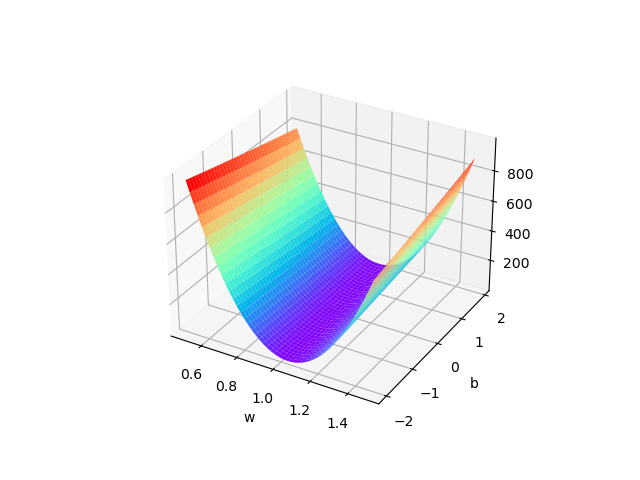# 深度学习01-线性回归入门

 1 import numpy as np
2 # 使用 Axes3D 的必要操作
3 import matplotlib.pyplot as plt
4
5 figure = plt.figure()
6 # ax = Axes3D(figure)
7 ax = figure.gca(projection="3d")
8
9 # 从文件读取数据
10 file = open('archive/test.csv')
12 x_data = []
13 y_data = []
14 while True:
16     if not text:
17         break
18     data = text.split(',')
19
20     x_data.append(float(data))
21     # 因为有'\n'所以要删掉才能转换为浮点数
22     y_data.append(float(data.rstrip('\n')))
23
24 file.close()
25
26 W = np.arange(0.5, 1.5, 0.01)
27 # bias只会影响图像的平移
28 B = np.arange(-2.0, 2.0, 0.1)
29 [w, b] = np.meshgrid(W, B)
30
31 # y = x-0.08
32 def forward(x):
33     return x * w + b
34
35 def loss(x, y):
36     y_pred = forward(x)
37     return (y_pred - y) * (y_pred - y)
38
39 # 损失求和
40 l_sum = 0
41 for x_val, y_val in zip(x_data, y_data):
42     l_sum += loss(x_val, y_val)
43
44 # 画 Surface3d：https://matplotlib.org/stable/tutorials/toolkits/mplot3d.html#surface-plots
45 ax.plot_surface(w, b, l_sum / len(x_data), cmap="rainbow")
46 plt.xlabel('w')
47 plt.ylabel('b')
48 plt.show()1、b 也就是bias 只会影响图像的上下平移，并不会影响拟合，所以这里不用加b ，直接找w 与loss 的关系即可。

2、读取数据时要注意数据的格式，下载的训练数据集train.csv 第215 行x 与y 中间没有',' 分隔，导致读取的时候总是报错。

1、读取文件的方式略微有点简陋，无伤大雅但是看到kaggle 上有前辈使用pandas 读取csv 文件只需要短短几行就可以将数据集读取出来，我还远远不足，需要勤加学习。

2、这个代码还是使用穷举法的思想，先想到w 和b 在什么范围，然后穷举的方式人工找出最好的拟合函数，暂时还不会如何训练出模型然后拟合测试集，需要勤加学习。

3、对python 语言还不够熟练，不太看得懂文档，画图的时候参考了前辈的文章：https://blog.csdn.net/weixin_44841652/article/details/105017087

posted @ 2021-04-25 16:38  RabbitKeeper  阅读(67)  评论(0编辑  收藏  举报